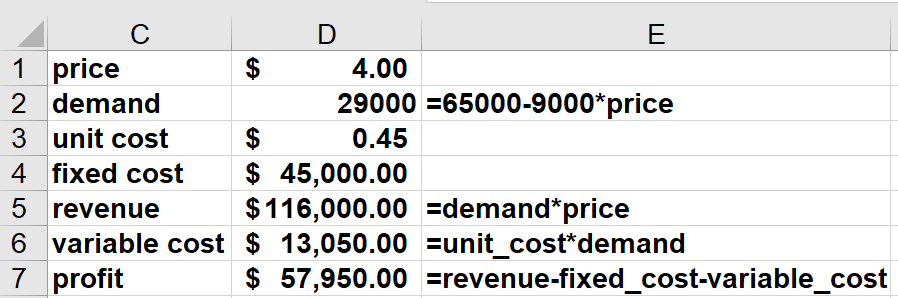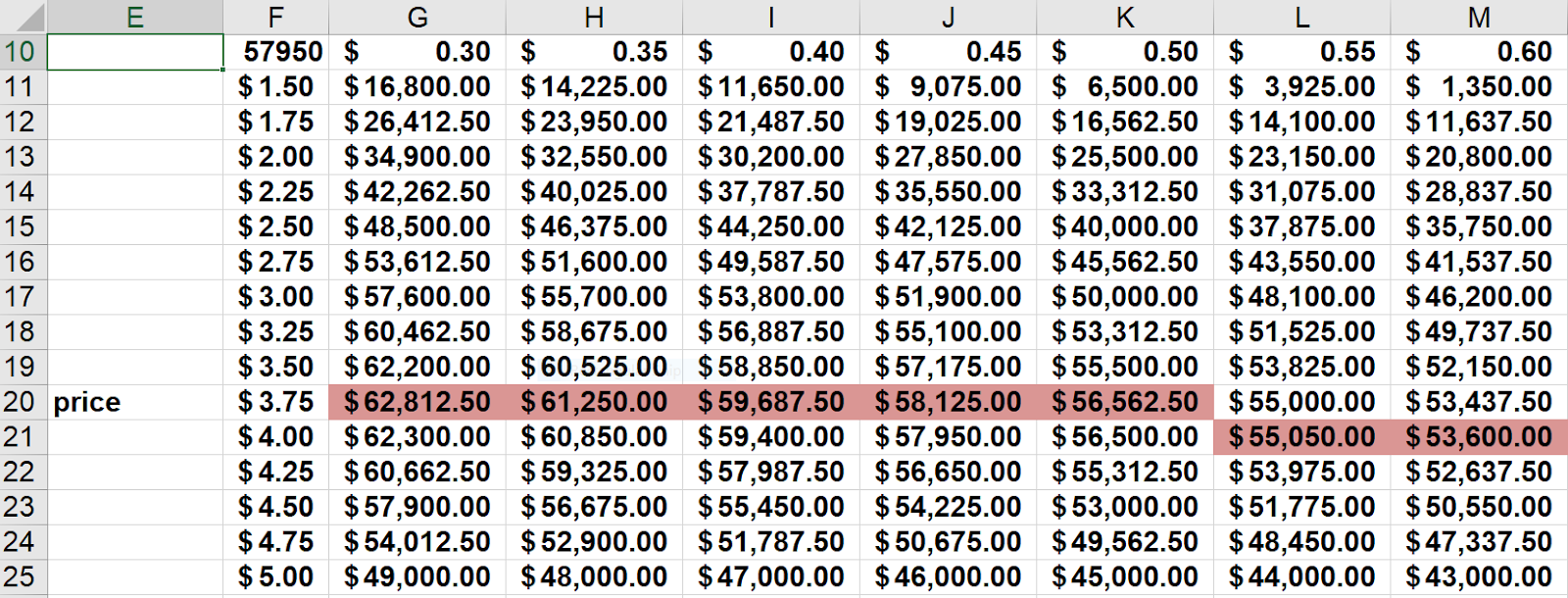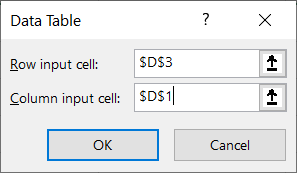# How to create sensitivity analysis in Excel with two-way data tablesAt some point in your accounting career, you may be asked to assess how your organization's revenue will be affected by specific input changes. In this case, a sensitivity table in Excel may prove useful. Sensitivity tables are dependent upon spreadsheet inputs and outputs. Spreadsheet inputs are assumptions that we make. For example, the price of a product might be a spreadsheet input. Spreadsheet outputs are quantities of interest that depend on the value of the spreadsheet’s inputs. For example, a company’s annual profit might be a spreadsheet output.

In this article, we explain how to use a two-way data table to explore how changes in two inputs affect a single spreadsheet output.

## Sensitivity analysis in Excel

The worksheet Coffee of the workbook BeckerJanuary2020.xlsx contains a simple model that computes annual profit at the Central Perk coffee shop. As shown in Figure 1 the annual demand for coffee is 65,000 -9000*price. This implies that an increase in the price of a cup of coffee will reduce annual sales by 9000 cups. In addition to the demand curve, the key inputs assumptions are the unit price (currently assumed to be \$4.00) and the unit cost of producing a cup of coffee (currently assumed to be \$0.45.) We want to determine how changes in the price and unit cost input affect profit.Figure 1 Central Perk’s Profit

Suppose Central Perk is considering a price of between \$1.50 and \$5.00 for a cup of coffee and they feel the unit cost will be between \$0.30 and \$0.60. To determine how variation in the price and unit cost affect profit we do the following:

1. Enter possible prices between \$1.50 and \$5.00 (in 25 cent increments) down a column (we chose F11:F25).
2. Move up one row and over to the right one column from our first price value(this takes us to G11) and enter in G11:M11 the unit cost values between \$0.30 and \$0.60 in five-cent increments.

The two-way data table shown in Figure 2 shows Central Perk’s profit for each price and unit cost. For example, in cell J20 the data table computes the profit when we charge \$3.75 and the unit cost is \$0.45 (\$58,125). To create the sensitivity analysis in Excel with a two-way data table we proceed as follows.

1. Enter the output cell (profit) in cell F10 by typing =Profit. The data table will recalculate Profit for each combination of price and unit cost.
2. Select the table range F10:M25. The table range contains the list of inputs, the output cell, and the cells where the output cell will be calculated for each combination of inputs.
3. From the Data Tab choose What-If Analysis from the Forecast Group and select Data Table.
4. Fill in the dialog box as shown in Figure 3. The row input cell is D3 because the numbers in the first row of the table range are unit costs and should be assigned to D3 and the column input cell is D1 because the numbers in the first column of the table range are prices, which should be assigned to cell D1.
5. After clicking OK, Excel knows to put each price in D1 and each unit cost in D3 and for each price-unit cost combination compute the output cell (profit.) In a matter of seconds, you have computed profit for 105 different price-unit cost scenarios.Figure 2 Two-way data sensitivity table for Central Perk's profitFigure 3 Data table dialog box

## Interpreting the sensitivity table in Excel

Examination of a sensitivity analysis in Excel will often give you important insights into how changing values of key assumptions influences profit. Here are possible insights:

1. Looking across each row of the data table we find that for a given price, an increase in unit cost reduces profit.
2. Looking down each column of the data table, we find that for a given unit cost, an increase in price will increase profit up to a certain point, but beyond a certain price, an increase in price will reduce profit. We have highlighted in pink the profit-maximizing price for each unit cost and you find, as expected, that as unit cost increases, the profit-maximizing price will remain the same or increase.

## Summarizing a sensitivity analysis in Excel with a chart

As the Chinese say, “a picture is worth 1000 words.” We can use a chart to illuminate the information contained in a data table. To summarize our sensitivity table with a chart proceed as follows:

1. Select the cell range F10:M25 and click on Control+C.
2. Go to cell F28 and right-click and choose the Paste Special Values Icon (the 123 button) to paste the information from the data table.
3. Delete the contents of cell F28.
4. Select the range F28:J43 and from the Insert Tab choose the Scatter with Smooth Lines Chart from the Scatter chart icon.
5. You will now see the chart shown in Figure 4. We see that for each price profit becomes larger as unit cost is reduced. We also find that at lower prices the curves for each unit cost are further apart. This is because at a lower price more units are sold, and an increase in unit cost will result in a larger decrease in profit.

By default, sensitivity tables in Excel recalculate whenever you change the spreadsheet. If you find that the recalculation of the sensitivity tables in a large spreadsheet annoys you, then from the Formulas tab go to Calculation Options and change the option to Automatic Except for Tables. Then your spreadsheets will not recalculate unless you hit the F9 key.

If you hit the Delete key when you are in any of the calculated cells in a data table, you will be told you can’t delete any part of a data table.

If you hover over any calculated cell in our data table, then you will see the formula ={TABLE(D3,D1). This formula tells you that the data table has Row input cellD3and the column input cell for the Data Table is D1.

Of course, you can create as many two-way sensitivity tables as needed. For example, if you had five inputs, you could create ten two-way tables: one for each set of two inputs.

I hope you will use two-way data tables to help you better understand the vast array of uncertainties present in your business environment.

### Now Leaving Becker.com

You are leaving the Becker.com website. Once you click “continue,” you will be brought to a third-party website. Please be aware, the privacy policy may differ on the third-party website. Adtalem Global Education is not responsible for the security, contents and accuracy of any information provided on the third-party website. Note that the website may still be a third-party website even the format is similar to the Becker.com website.